# KSEEB SSLC Class 10 Maths Solutions Chapter 15 Surface Areas and Volumes Ex 15.1

KSEEB SSLC Class 10 Maths Solutions Chapter 15 Surface Areas and Volumes Ex 15.1 are part of KSEEB SSLC Class 10 Maths Solutions. Here we have given Karnataka SSLC Class 10 Maths Solutions Chapter 15 Surface Areas and Volumes Exercise 15.1.

## Karnataka SSLC Class 10 Maths Solutions Chapter 15 Surface Areas and Volumes Exercise 15.1

(Unless stated otherwise, take π $$=\frac{22}{7}$$)

Question 1.
2 cubes each of volume 64cm3 are joined end to end. Find the surface area of the resulting cuboid.
Solution:
If measurement of each side of each cube be l cm, then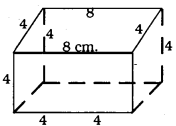(l)3 $$=\sqrt{64}$$
∴ l = 4 cm.
Length of cubes if both joined together,
Length, l = 4 + 4 = 8 cm.
height, h = 4 cm.
Surface area of the cuboid,
= 2lb + 2lh + 2bh
= 2(8 × 4) + 2(8 × 4) + 2(4 × 4)
= 2 × 32 + 2 × 32 + 2 × 16 = 64 + 64 + 32
= 160 sq.cm.

Question 2.
A vessel is in the form of a hollow hemisphere mounted by a hollow cylinder. The diameter of the hemisphere is 14 cm and the total height of the vessel is 13 cm. Find the inner surface area of the vessel.
Solution:
(i) Diameter of a hollo hemisphere = 14 cm.
∴ Radius of a hollow hemisphere, r
r = 7 cm.
Inner curved surface of a hemisphere = 2π2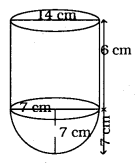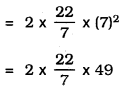= 14 × 22
= 308 sq. cm.
(ii) Radius of hollow cylinder, r = 7 cm.
Height, h = 13 – 7 = 6 cm.
∴ Inner Surface area of the Cylinder,
= 2πrh
$$=2 \times \frac{22}{7} \times 7 \times 6$$
= 44 × 6
= 264 sq.cm.
∴ Inner surface area of the vessel = Area of hemisphere + Area of cylinder
= 308 + 264
= 572 sq. cm.

Question 3.
A toy is in the form of a cone of radius 3.5 cm mounted on a hemisphere of same radius. The total height of the toy is 15.5 cm. Find the total surface area of the toy.
Solution:
(i) Radius of base of circular toy,
r = 3.5 cm.
∴ Surface Area of hemisphere, = 2πr2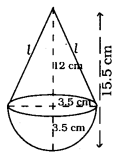= 77 sq.cm
(ii) Let slant height of cone is l cm. then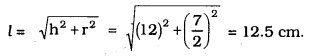∴ Curved surface area of a cone = πrl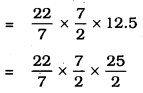= 137.5 sq.cm.
∴ Total surface area of a toy = Surface area of hemisphere + Curved surface area of a cone
= 77.0 + 137.5
= 214.5 sq.cm.

Question 4.
A cubical block of side 7 cm is surmounted by a hemisphere. What is the greatest diameter the hemisphere can have ? Find the surface area of the solid.
Solution:
(i) A cubical block of side 7 cm is surmounted by a hemisphere.
∴ Diameter of hemisphere is = 7 cm.
Radius of hemisphere, r = 3.5 cm.
∴ Surface area of hemisphere = Curved surface area – Area of base of hemisphere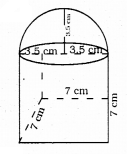= 38.5
(ii) The curved surface area of square,
=6 × l2
= 6 × (7)2
= 6 × 49
= 294 sq. cm.
∴ Total surface area of cubical block, = Curved surface area of cubical block + Surface area of hemisphere.
= 294 + 38.5
=332.5sq.cm.

Question 5.
A hemispherical depression is cut out from one face of a cubical wooden block such that the diameter l of the hemisphere is equal to the edge of the cube. Determine the surface area of the cube. Determine the surface area of the remaining solid.
Solution:
Let, Length of each edge of cubical block = Diameter of hemisphere = l
∴ Radius of hemisphere, $$r=\frac{l}{2} unit$$.Total surface area of newly formed cube = Curved surface area of cube – Upper part of Cube + Area of hemisphere which is depressed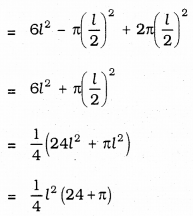Question 6.
A medicine capsule is in the shape of a cylinder with two hemispheres stuck to each of its ends (see Figure given below). The length of the entire capsule is 14 mm and the diameter of the capsule is 5 mm. Find its surface area.Solution: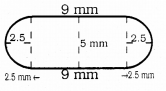(i) Surface area of cylindrical capsule, = 2πrh
$$=2 \pi \times\left(\frac{5}{2}\right) \times 9$$
= 45π mm2,
(ii) Surface area of both hemispheres,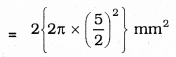= 25π mm2
∴ Total surface area of capsule, = 45π + 25π mm2
= 70π mm2
$$=70 \times \frac{22}{7}$$
= 220 mm2

Question 7.
A tent is in the shape of a cylinder surmounted by a conical top. If the height and diameter of the cylindrical part are 2.1m and 4m respectively, and the slant height of the top is 2.8 m, find the area of the canvas used for making the tent. Also, find the cost of the canvas of the tent at the rate of Rs. 500 per m2. (note that the base of the tent will not be covered with canvas.)
Solution:
(i) Radius of base of cylinder, r= 2 mm.
Height of base of cylinder, h= 2.1 m.
∴ Curved Surface area of cylinder = 2πrh
= 2π × 2 × (2.1)
$$=4 \times \frac{22}{7} \times 2.1$$
= 26.4 m2.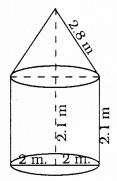r = 2 m.
length, l = 2.8 m.
∴ Curved Surface area of cylinder = πrl
$$=\frac{22}{7} \times 2 \times 2.8$$
= 17.6 m2.
∴ Total area of the tent = 26.4 + 17.6
= 44m2
Cost of 1 aq.m. of canvas is Rs. 500.
Cost of 44 sq. m. canvas … ??
500 × 44
= Rs. 22,000.

Question 8.
From a solid cylinder whose height is 2.4 cm and diameter 1.4 cm, a conical cavity of the same height and same diameter is hollowed out. Find the total surface area of the remaining solid to the nearest cm2.
Solution:
Height of the cylinder, h = 2.4 m.
Diameter of the cylinder, d = 1.4 m.
∴ Radius, r = 0.7 m.
(i) Outer Curved surface area of cylinder = 2πrh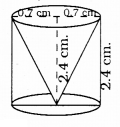$$=2 \times \frac{22}{7} \times(0.7) \times 2.4$$
= 44. × 0.24
= 10.56 cm2.
(ii) Area of base of = πr2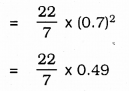= 1.54 cm2.
(iii) Inner surface area of cylinder, = πrl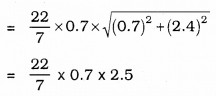= 5.5 cm2
∴ Total surface area of newly formed cube = 10.56 + 1.54 + 5.5 = 17.6 cm2.
∴ Nearest value is 18 sq.cm.

Question 9.
A wooden article was made by scooping out a hemisphere from each end of a solid cylinder, as shown in the figure. If the height of the cylinder is 10 cm. and its base is of radius 3.5 cm. find the total surface area of the article.Solution:
Radius of base of cylinder, r= 3.5 cm
Height, h = 10 cm.Total area of article, = Curved Surface area of Cylinder + 2 × Area of Hemisphere
= 2πrh + 2 × 2πr2= 374 sq.cm.

We hope the given KSEEB SSLC Class 10 Maths Solutions Chapter 15 Surface Areas and Volumes Ex 15.1 will help you. If you have any query regarding Karnataka SSLC Class 10 Maths Solutions Chapter 15 Surface Areas and Volumes Exercise 15.1, drop a comment below and we will get back to you at the earliest.

error: Content is protected !!